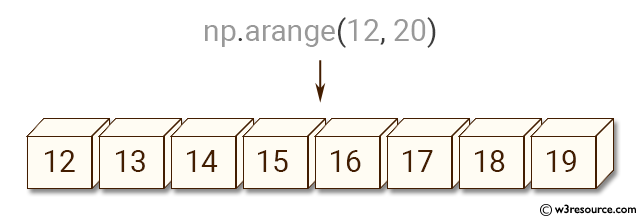﻿ NumPy: Create an array with values ranging from 12 to 38 - w3resource# NumPy: Create an array with values ranging from 12 to 38

## NumPy: Array Object Exercise-5 with Solution

Write a NumPy program to create an array with values ranging from 12 to 38.Sample Solution:-

Python Code:

``````import numpy as np
x = np.arange(12, 38)
print(x)
```
```

Sample Output:

```[12 13 14 15 16 17 18 19 20 21 22 23 24 25 26 27 28 29 30 31 32 33 34 35
36
37]
```

Python Code Editor:

Have another way to solve this solution? Contribute your code (and comments) through Disqus.

What is the difficulty level of this exercise?

Test your Python skills with w3resource's quiz

﻿

## Python: Tips of the Day

Set comprehension:

```>>> m = {x ** 2 for x in range(5)}
>>> m
{0, 1, 4, 9, 16}
```